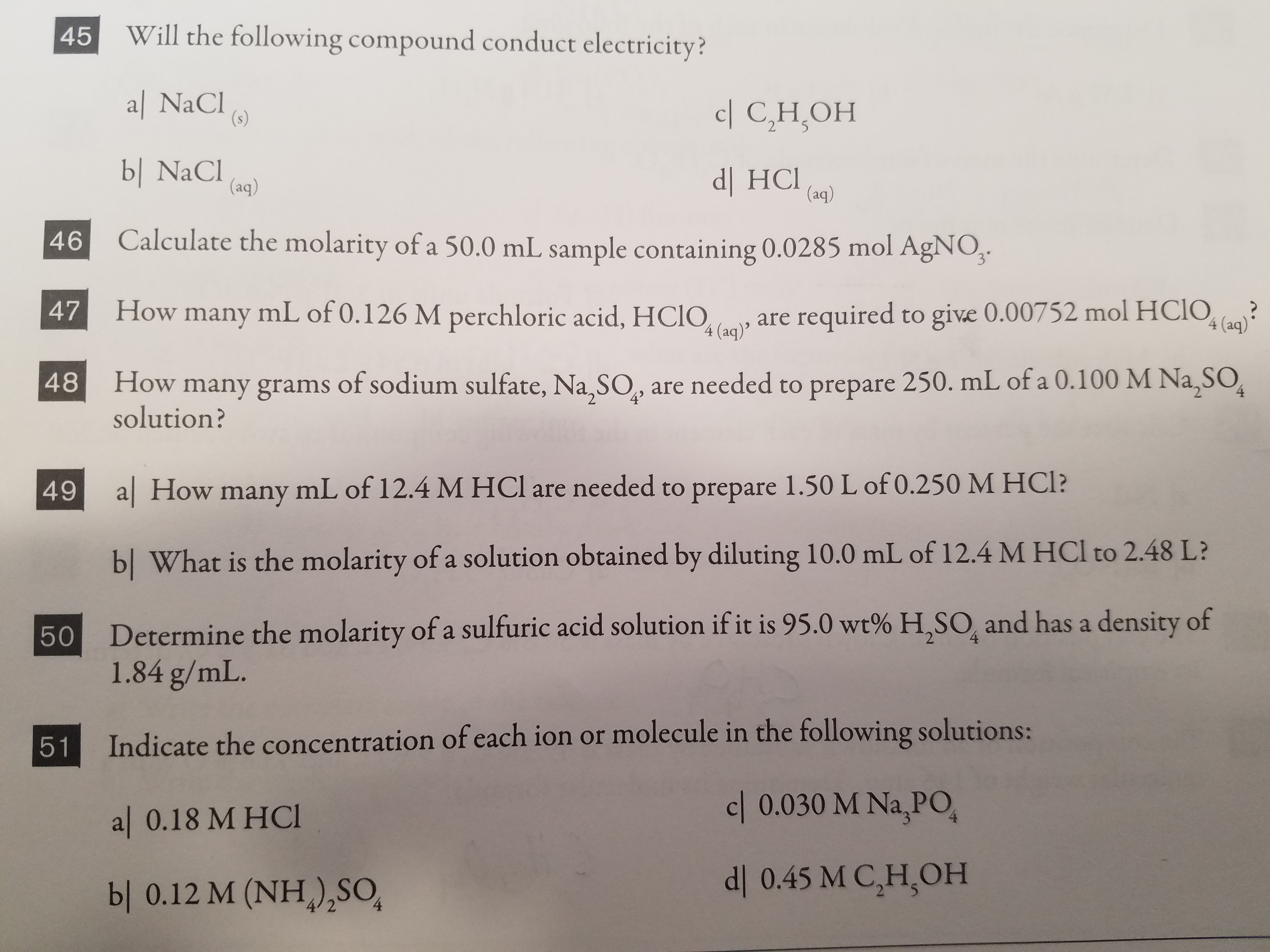# 45 Will the following compound conduct electricity?W'ill the following compound conduct electricityal NaCIbl NaCICalculate the molarity of a 50.0 mL sample containing 0.0285 mol AgNOHow many mL of 0.126 M perchloric acid, HCIOquired to give 0.00752 mol HCOc| C,H,OHdl HCl)46C48 How many grams of sodium sulfate, Na,SO, are needed to prepare 250. mL of a 0.100 M Na,SO49 a| How many mL of 12.4 M HCl are needed to prepare 1.50 L of 0.250 M HCI474 (aq)solution?bl What is the molarity of a solution obtained by diluting 10.0 mL of 12.4 M HCl to 2.48 L?Determine the molmolarity of a sulfuric acidsolution if it is95.0 wt% H,SO and has a density of1.84 g/mL.Indicate the concentration of each ion or molecule in the following solutional 0.18 M HCIbl 0.12 M (NH) SO51c| 0.030 M Na,POdl 0.45 M C,H,OH

Question
6 viewshelp_outlineImage Transcriptionclose45 Will the following compound conduct electricity? W'ill the following compound conduct electricity al NaCI bl NaCI Calculate the molarity of a 50.0 mL sample containing 0.0285 mol AgNO How many mL of 0.126 M perchloric acid, HCIOquired to give 0.00752 mol HCO c| C,H,OH dl HCl) 46C 48 How many grams of sodium sulfate, Na,SO, are needed to prepare 250. mL of a 0.100 M Na,SO 49 a| How many mL of 12.4 M HCl are needed to prepare 1.50 L of 0.250 M HCI 47 4 (aq) solution? bl What is the molarity of a solution obtained by diluting 10.0 mL of 12.4 M HCl to 2.48 L? Determine the mol molarity of a sulfuric acid solution if it is 95.0 wt% H,SO and has a density of 1.84 g/mL. Indicate the concentration of each ion or molecule in the following solution al 0.18 M HCI bl 0.12 M (NH) SO 51 c| 0.030 M Na,PO dl 0.45 M C,H,OH fullscreen
check_circle

Step 1

Hey, since there are multiple questions posted, we will answer first question. If you want any specific question to be answered then please submit that question only or specify the question number in your message.

(a)

Sodium chloride will not conduct electricity in its solid form.

Step 2

(b)

Sodium chloride will conduct electricity in its aqueous form.

Step 3

(c)

Ethanol is an extremely poor conductor of...

### Want to see the full answer?

See Solution

#### Want to see this answer and more?

Solutions are written by subject experts who are available 24/7. Questions are typically answered within 1 hour.*

See Solution
*Response times may vary by subject and question.
Tagged in

### Electrochemistry